## Combination Lock

Let a combination ofbuttons be a Sequence of disjoint nonempty Subsets of the Set. If the number of possible combinations is denoted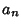, thensatisfies the Recurrence Relation(1)

with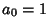. This can also be written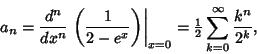(2)

where the definition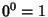has been used. Furthermore,(3)

whereare Eulerian Numbers. In terms of the Stirling Numbers of the Second Kind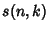,(4)can also be given in closed form as(5)

whereis the Polylogarithm. The first few values offor, 2, ... are 1, 3, 13, 75, 541, 4683, 47293, 545835, 7087261, 102247563, ... (Sloane's A000670).

The quantity(6)

satisfies the inequality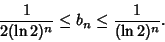(7)

References

Sloane, N. J. A. Sequence A000670/M2952 in An On-Line Version of the Encyclopedia of Integer Sequences.'' http://www.research.att.com/~njas/sequences/eisonline.html and Sloane, N. J. A. and Plouffe, S. The Encyclopedia of Integer Sequences. San Diego: Academic Press, 1995.

Velleman, D. J. and Call, G. S. Permutations and Combination Locks.'' Math. Mag. 68, 243-253, 1995.